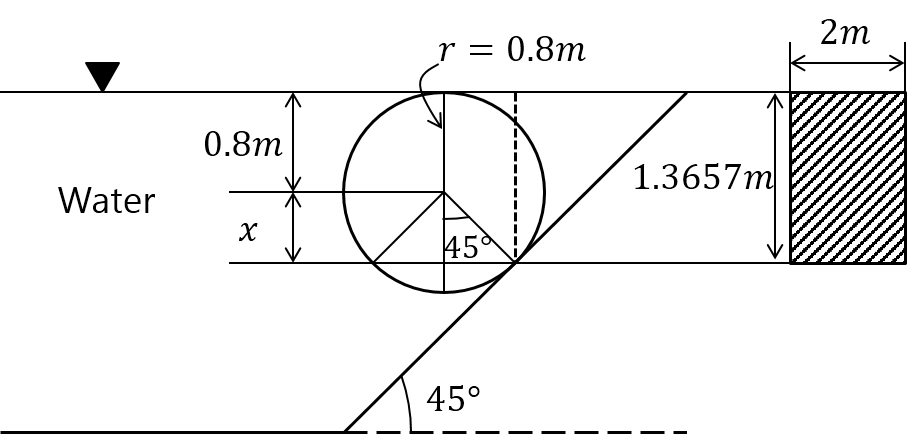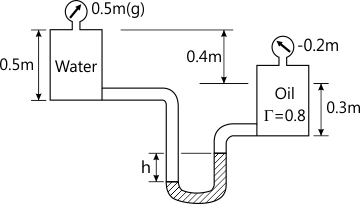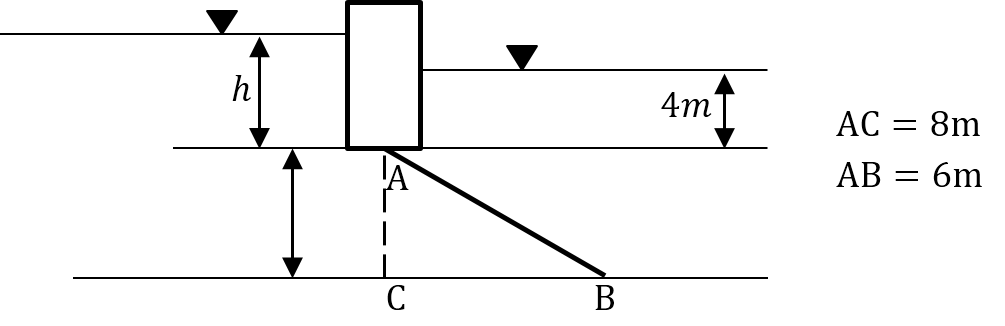MU Mechanical Engineering (Semester 4)
Fluid Mechanics
December 2013
Total marks: --
Total time: --
INSTRUCTIONS
(1) Assume appropriate data and state your reasons
(2) Marks are given to the right of every question
(3) Draw neat diagrams wherever necessary

1 (a) A two-dimensional flow field is given by u=2y2; v = 3x. Find the acceleration at point A (-2,4).
6 M
1 (b) Pressurized water fills the tank and hemispherical dome given in the figure below. Compute the net hydrostatic force of water on the dome.
6 M
1 (c) Does the following potential represent possible flow? If so determine the stream function and plot the streamline Ψ=1.
Φ=y+ x2- y2
6 M
1 (d) Convert 12560 Pascal's (absolute) into mm of mercury gauge.
2 M

2 (a) The cylinder shown in the figure is 2m wide. Compute the horizontal and vertical components of the force due to water on the cylinder.10 M
2 (b) A reducing bend in a horizontal plane as shown below. The diameter at the inlet A is 100mm and at exit B is 50mm. If the pressure at inlet is 0.5 kg/cm2(gauge) and the flow rate is 1200 liters/min. Calculate the net force on the bend due to change in momentum of the fluid, which is water. Assume frictionless flow. Use the equations for an integral control volume to solve the problem.
10 M

3 (a) Oil flows through a pipeline of 0.4 diameter. The flow is laminar and the velocity at any radius is given by U= (0.6 − 15r2) m/s. calculate the volume flow rate, the average velocity and the momentum correction factor.
10 M
3 (b) A flow has a velocity potential function is given by φ=x3-3xy2. Verify whether it represents a valid flow field. If it does then determine the stream function and calculate the velocity and pressure at (1,-3) given that the pressure at (4, 1) is 14.5 KPa and the fluid is water.
10 M

4 (a) The Darcy-weisbach equation for head loss through a pipe is given as
hf=(flv2)/2gd
A reservoir stores water to a height of 12m. A horizontal pipe of 10cm diameter and 50m length, connected at the base of the reservoir, carries water from the reservoir. The entrance to the pipe is sharp with a loss co-efficient of 0.5 and the pipe has a friction factor of 0.018. Calculate the discharge through the pipe for steady conditions. If a nozzle of 50mm outlet diameter and a cv value of 0.92 is connected to the exit of the pipe., by what percentage does the discharge vary, assuming other conditions to be the same.
14 M
4 (b) Define stream function and velocity potential function. Prove that streamlines and equipotential lines are normal to each other in a flow field.
6 M

5 (a) For the U tube manometer shown in the diagram below calculate the difference in the mercury column in the two limbs.8 M
5 (b) Gate is 7m wide and weighs 30,000 N when submerged. It is hinged at B and rests against a wall a smooth wall at A. Determine the water level 'h' at the left which will cause the gate to open.12 M

6 (a) With the help of a neat diagram discuss the phenomenon of boundary layer separation over a curved surface and explains the concept of form drag. What are streamlined bodies and bluff bodies? Explain with reference to their drag coefficients.
6 M
6 (b) Draw a sketch of a venturimeter and derive an expression for the discharge through the venturimeter. Explain the terms CV, CC, Cd.
12 M
6 (c) What is CFD? What are the benefits of CFD simulation? Where it is used?
2 M

7 (a) State and explain Prandtl's mixing length hypothesis and derive an expression for turbulent stresses. Using this expression derive the universal velocity profile for a turbulent flow in a pipe.
10 M
7 (b) A doublet at the origin is combined with a uniform rectilinear flow of 12m/s along the positive X axis to give flow past a cylinder of diameter of 4 m. Calculate the strength of the doublet. A clockwise vortex of strength 150 m2/s is superimposed on this flow at the centre of the cylinder. Calculate the location of the stagnation points. What is the pressure on the cylinder surface at θ=90° if the pressure far upstream is negligible.
10 M

More question papers from Fluid Mechanics